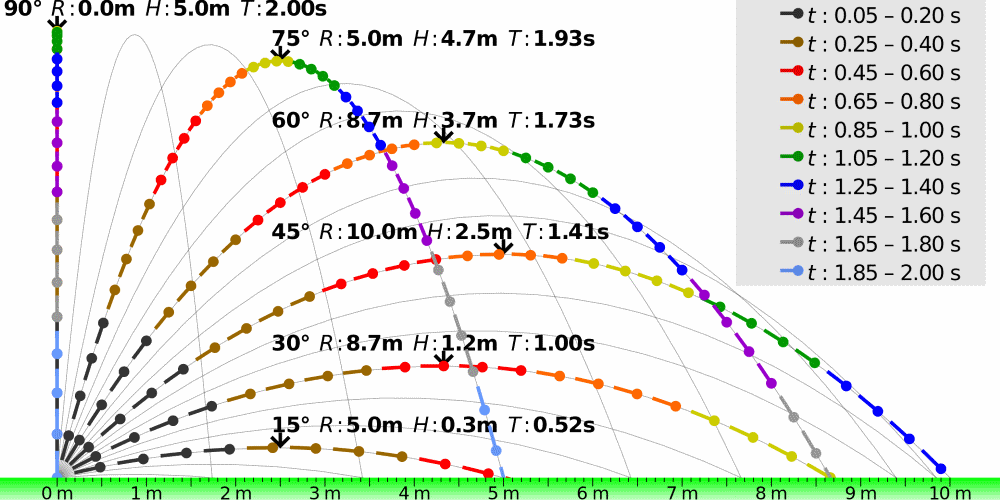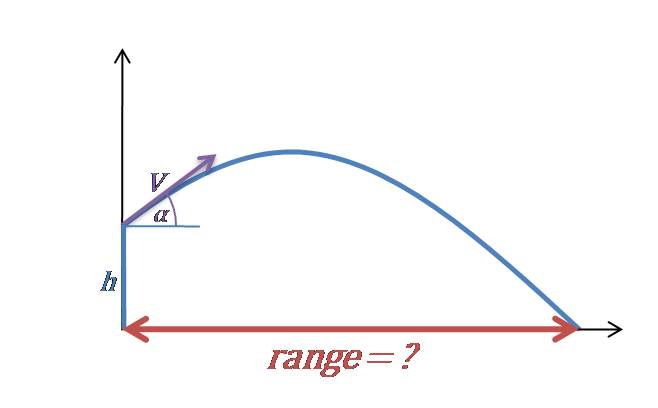# Projectile Range Calculator

Created by Hanna Pamuła, PhD
Reviewed by Bogna Szyk and Steven Wooding
Last updated: Jan 04, 2023

With this projectile range calculator, you'll quickly find out how far you can throw the object. All you need to do is enter the three parameters of projectile motionvelocity, angle, and height from which the projectile is launched. In no time, you'll find the horizontal displacement of your object. Experiment with the calculator and discover which angle guarantees a projectile's maximum distance – or scroll down and learn more about projectile range formulas.

🔎 If you want to find the range for horizontal speed only (so angle = 0), you can use this projectile range calculator or go directly to the horizontal projectile motion calculator page. Air resistance is neglected in all calculations.

## Projectile range formulas

Let's split the equations into two cases: when we launch the projectile from the ground and when the object is thrown from some initial height (e.g., table, building, bridge).

1. Launch from the ground (initial height = 0)

To find the formula for the projectile range, let's start with the equation of motion. The projectile range is the distance traveled by the object when it returns to the ground (so y = 0):

0 = V₀ × t × sin(α) - g × t²/2

From that equation, we'll find t, which is the time of flight to the ground:

t = 2 × V₀ × sin(α)/g

Also, we know that we can find the maximum distance of the projectile from the widely known formula: d = V × t (learn more in our distance calculator). Velocity, in our case, is the horizontal velocity Vx = V₀ × cos(α), and the time to reach the ground is a value we've already calculated:

d = V × t = V₀ × cos(α) × 2 × V₀ × sin(α)/g

d = 2 × V₀² × cos(α) × sin(α)/g

If you've used the trigonometric functions calculator, you know that sin(2x) = 2sin(x)cos(x), so we can write the final formula as:

d = V₀² × sin(2 × α)/gTrajectories of projectiles launched at different elevation angles but at the same speed (g = 10 m/s²). Attribution: Cmglee, CC BY-SA 3.0, via Wikimedia Commons.

Did you find out when distance is maximal? Yes, it's when the sin(2α) term has the highest possible value:

sin(2α) = 1 => 2α = 90° => α = 45°

2. Launch from an elevation (initial height > 0)

In that case, the time spent flying upwards is shorter than the time when the object is falling down. The formula for the projectile range is the same: the horizontal velocity (V₀) multiplied by the time of flight equation (launching from an initial height):

$\scriptsize \!d \!=\! V_0\cos \alpha \!\times\! \frac{V_0\sin \alpha \!+\! \sqrt{\!\left(\!V_0\sin \alpha\right)^2 \!\!+\! 2g h}}{g}$

## Projectile range calculator example

Let's find out the range of the hypothetical volcanic ballistic projectiles 🌋:

1. Enter the projectile velocity. Assume a rock was ejected from the volcano with a speed of 30 m/s.
2. Type in the angle. Let's say that the angle was equal to 25°.
3. Fill in the box of initial height. Our volcano was relatively small, and the rock landed on the side of the volcano at a 100 m lower altitude than the launching point.
4. The projectile range calculator shows the answer! The maximal distance is 162.87 m for our volcano example. That's a lot!

In reality, projectile motion is a much more complicated phenomenon. The air resistance reduces a projectile's range – dependent on the object's volume, shape, surface smoothness, mass, etc.

## FAQ

### What is range in projectile motion?

The projectile range is the distance the object will travel from when you fire it until it returns to the same height at which it was fired. Note that no acceleration is acting in this direction, as gravity only acts vertically. To determine the projectile range it is necessary to find the initial velocity, angle, and height. We usually specify the horizontal range in meters (m).

### How can I calculate projectile range?

Imagine that a footballer kicked the ball from ground level at an angle of 35° with an initial velocity of 22.0 m/s. To find the projectile range:

1. Take the initial velocity: V₀ = 22 m/s.
2. Identify the angle: α = 35°.
3. Assume that the initial height = 0.
4. Enter data into the equation:
d = V₀² × sin(2 × α)/g =
= (22 m/s)² × sin(2 × 35°)/9.81 m/s²
= 46.4 m
.

The ball landed 46.4 m from the player.

### What is the condition for minimum range of projectile?

The projection range will be minimum if the angle of projection is 90°. Note that sin(2×90°) = sin(180°) = 0 and therefore d = V₀² × sin(180°)/g = 0. On the other hand, the maximum range will be at an angle of 45°.

### Does range of projectile depend on mass?

No, mass does not affect the projectile's motion, and its value is irrelevant. In projectile motion, parameters such as distance traveled, time taken by the projectile, and maximum height depend only on the initial velocity, height, and the angle of the throw.

### What is the projectile range of a basketball player 2 m tall when throwing a ball?

Assuming that the ball was thrown with a velocity of 8.2 m/s and an angle of 45°, the projectile range is 8.48 m. To calculate this:

1. Identify the initial height = 2 m, α = 45° and V₀ = 8.2 m/s.

2. Use the formula:
d = V₀cosα × (V₀sinα+√[(V₀sinα)²+2gh])/g =

8.2 m/s × cos45° × (8.2 m/s×sin45° + √[(8.2 m/s × sin45°)² + 2 × 9.81 m/s × 2 m])/9.81 m/s = 8.48 m.

Hanna Pamuła, PhD
Velocity
ft/s
Angle of launch
deg
Initial height
ft
Range
Range
ftPeople also viewed…

### Newton's law of cooling

This Newton's law of cooling calculator lets you find the time it takes for different objects to cool down.

### Pulley

Pulley calculator finds all parameters of a belt drive system: the RPM, speed, belt length, belt tension, and torque.